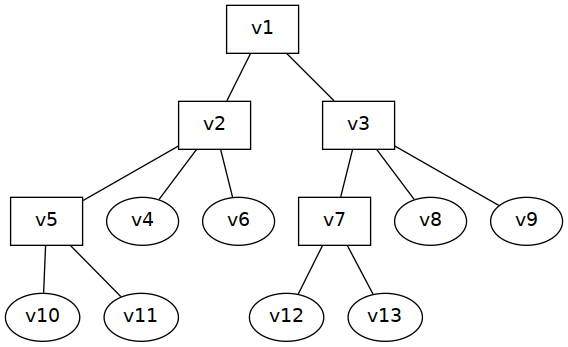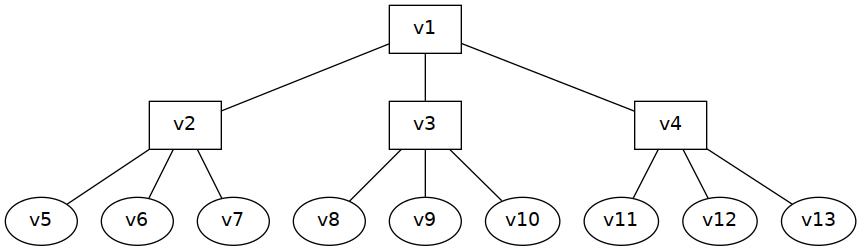Theory

Definition. A hierarchy is a single-rooted tree.

Let $$T=(V,E)$$ be a connected, acyclic directed graph that expresses a hierarchical structure. The set of vertices $$V$$ represents the entities that are being organized, and the set of edges $$E$$ maps from a given entity to its respective superior.A vertex is said to have degree $$k$$ if it has exactly $$k$$ direct subordinates (incoming edges). Vertices with degree 0 are called leaves and vertices with degree $$>0$$ are called nodes.

Definition. The average degree of a hierarchy is the average degree of its nodes.

If $$T$$ is not empty, then there exists exactly one distinguished vertex $$v_1\in{}V$$ that has no superior. All other vertices have exactly one superior.

For every $$v\in{}V$$ there exists a unique path that leads from $$v$$ to $$v_1$$. The length of that path is referred to as depth of $$v$$. The depth of $$v_1$$ is 0.

Definition. The average depth of a hierarchy is the average depth of its leaves.

Let $$\mu$$ be the average degree of $$T$$, and let $$l$$ be the average depth of $$T$$. Then there exists a perfectly regular tree $$T'$$ in which all nodes have degree $$\mu$$ and all leaves are at depth~$$l$$:Such a tree has $$\mu^k$$ vertices at any given depth $$k$$, which means that the total number of vertices is $n \;=\; \sum\limits_{k=0}^{l}\,\mu^k \;=\; \frac{1-\mu^{l+1}}{1-\mu} .$

Corollary. In every perfectly regular hierarchy, the relationship between the average degree $$\mu$$, the average depth $$l$$, and the number of vertices $$n$$ is $n\,\mu^{l+1}-\mu^{l}-n+1\;=\;0 .$

Corollary. The proportion of nodes in every perfectly regular hierarchy is $\frac{1-\mu^{l}}{1-\mu^{l+1}} .$

Application

Consider any given company hierarchy of $$n=10\,000$$ employees. Nodes represent managers and leaves represent workers. Workers have an average chain of $$l=4$$ superiors up to (and including) the big boss. If that hierarchy is somewhat regular, then approximately 10% of the workforce — $$1\,000$$ employees — would be required for purposes of management. The average team in that company would have approximately $$\mu=9,7$$ members. If the company match these predictions, then its hierarchy would have to be somewhat irregular.